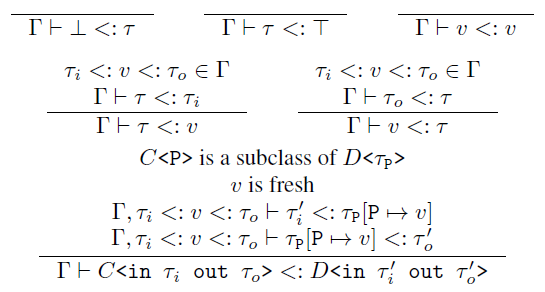The formal subtyping rules and algorithm for use-site variance

# Formalism Tutorial

In programming-languages research, we use a special language of sorts for formalizing languages and algorithms. This is a quick tutorial on that language for all the non-academics. This should help you read the paper Mixed-Site Variance by Ross Tate, among other academic publications.

## Symbols

First, let me break down the Greek a bit. Γ is generally used to denote contexts. In this case, it specifies lower and upper (subtyping) bounds on type variables, which I'm denoting with v. τ is generally used to denote types. Here, we have special types ⊥ (bottom) and Τ (top), which in the context of subtyping generally denote the subtype of everything and the supertype of everything respectively. The symbol "<:" is a common notation for the subtype relation.

## Judgements

Often a typing judgement is of the form "(context) |- (property)"; the "|-" symbol is read as "entails". In our case, the judgement looks like "Γ |- τ1 <: τ2", expressing the (not necessarily true) statement "with the constraints on type variables specified in Γ, the type τ1 is a subtype of τ2".

## Rules

In the picture, there are all these things of the form "(some number of judgements) over a long bar over (some judgement)". These are called rules and read as "if everything above the bar (the premises) is true, then the thing below the bar (the conclusion) is true"; any variable symbols used (e.g. Γ, τ, and v) are implicitly universally quantified. So the first rule says that ⊥ is a subtype of any type τ under any context Γ, unconditionally since there is nothing above the bar. Similarly the second rule says that every type is a subtype of Τ under any context, and the third rule says every type variable is a subtype of itself. Moving on, the fourth rule says that a type τ is a subtype of a type variable v in context Γ if τ is a subtype of the lower bound τi of v specified by Γ. The fifth rule is similar. The last rule I talk about in the paper, but let me explain some notation and vocabulary. The statement "v is fresh" means that v does not occur anywhere in the types being compared or in the context; it makes sure we do not accidentally use a variable name that already has some meaning. The notation τ[P |-> τ'] represents the result of substituting (i.e. replacing) all the uses of type variable P in type τ with type τ'.

## Algorithm

Specifications of subtyping systems often have rules for transitivity and subtyping. Here I have made those properties that can be proved about the system (under certain assumptions about Γ). The reason is that I wanted my formalization to be syntax directed. Notice that, for every rule, every variable used above the bar is determined by the values/contents of the variables below the bar, or the specific value of that variable doesn't matter (e.g. the fresh variable v) or is determined by some global information (e.g. τP is determined by the inheritance hierarchy). This fact actually gives you an algorithm for determining if two types are subtypes of each other (in some context). First, given a goal you want to prove, check which (if any) of the rules' conclusion matches your goal. Then for each of the variables in the premises, determine its value using the fact the rule is syntax directed. Finally, for each subytyping premise recursively repeat this pattern-matching process. Using this trick you get an algorithm for subtyping from the formal rules I presented. In the paper, you can extend this trick to get algorithms for tight approximation, though you have to be a little fancier because this time there are input and output variables.